Case Based Questions (MCQ)

Chapter 7 Class 12 Integrals
Serial order wise

## It is very useful because it gives us a method of calculating the definite integral more easily. There is no need to keep integration constant C because if we consider F(x)+C instead of F(x).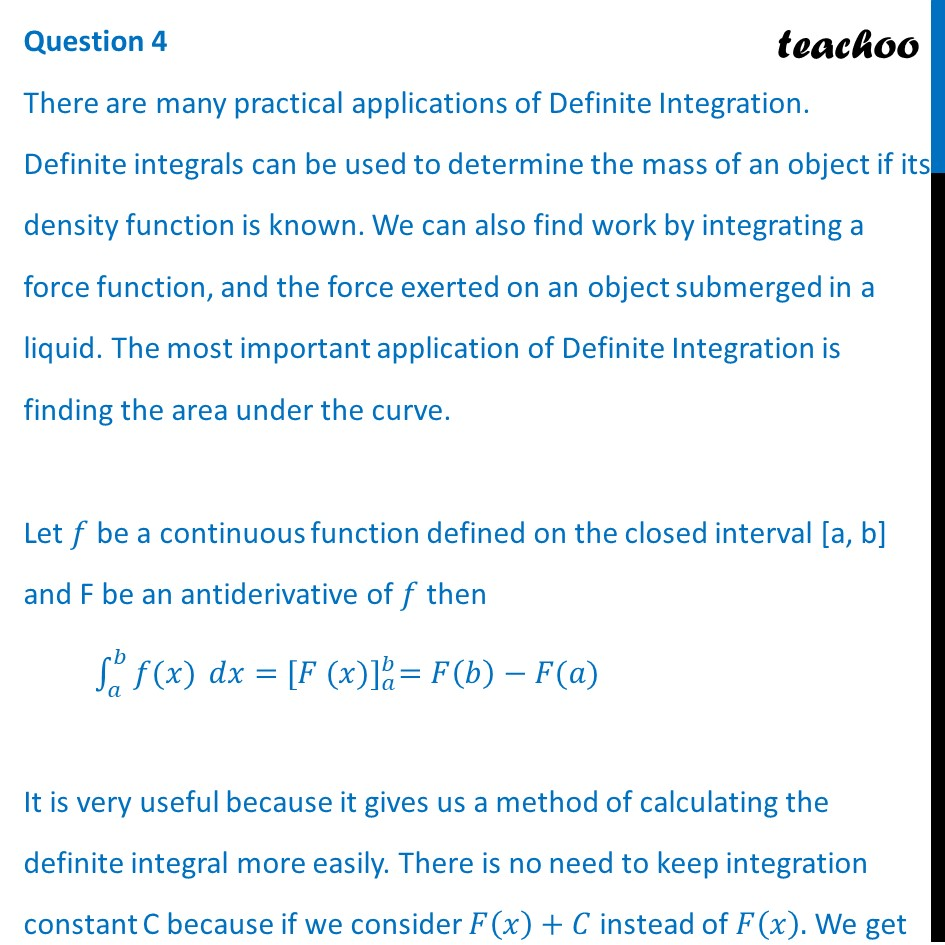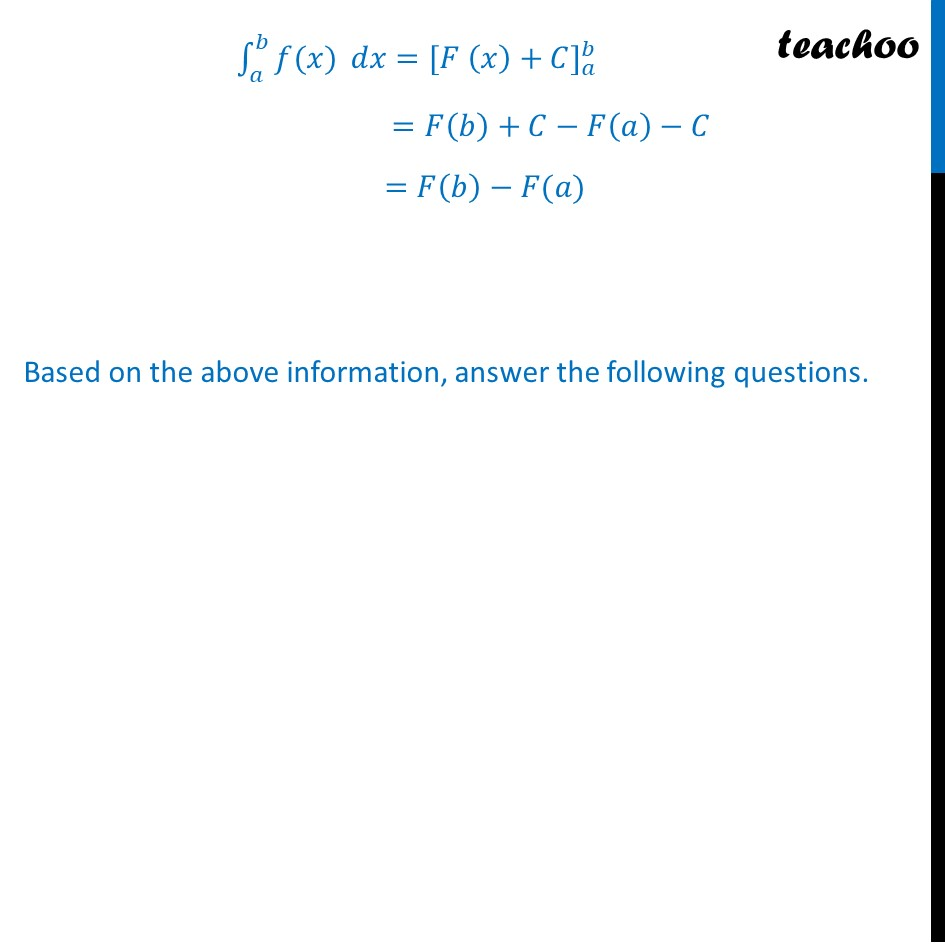## (d) 1/3

This question is inspired from Question 30 - CBSE Class 12 - Sample Paper for 2020 Boards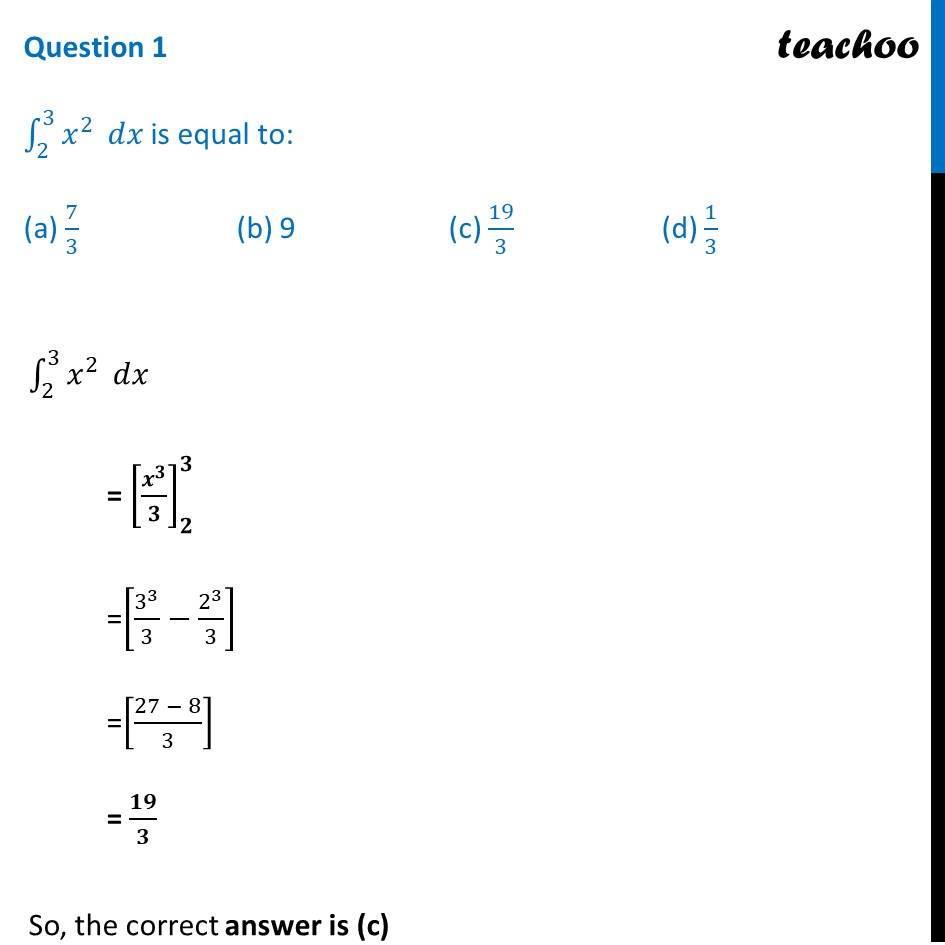## (d) π/12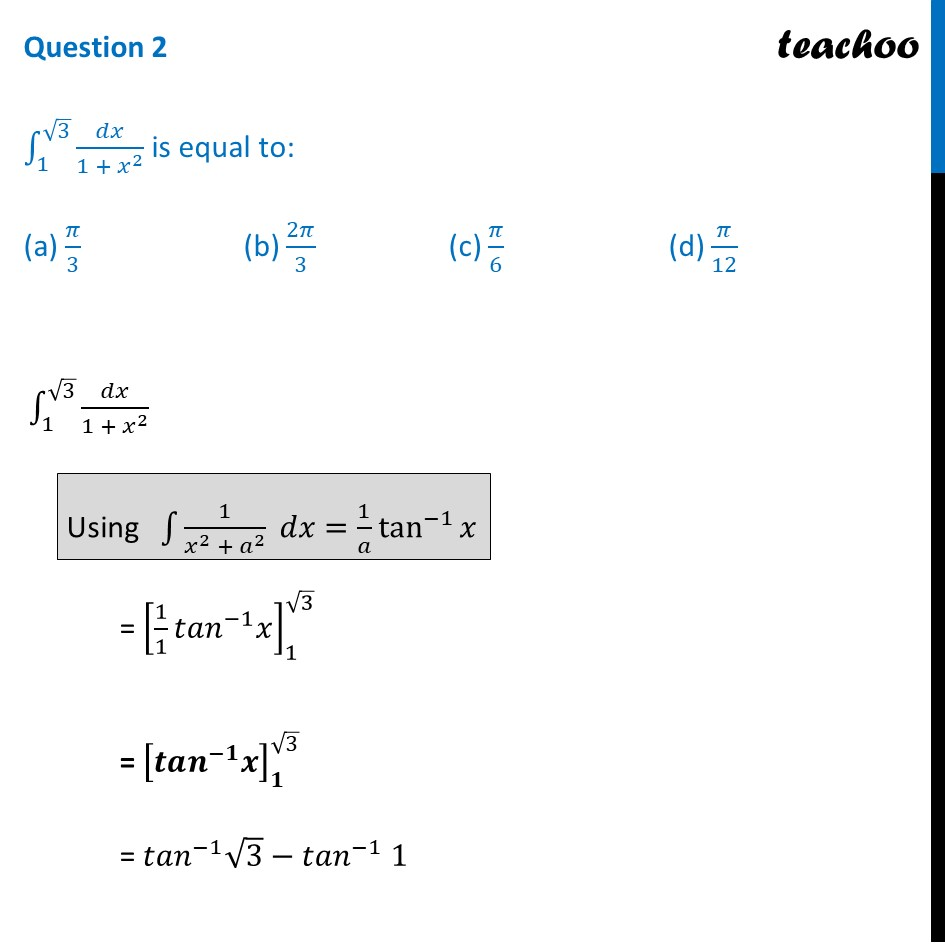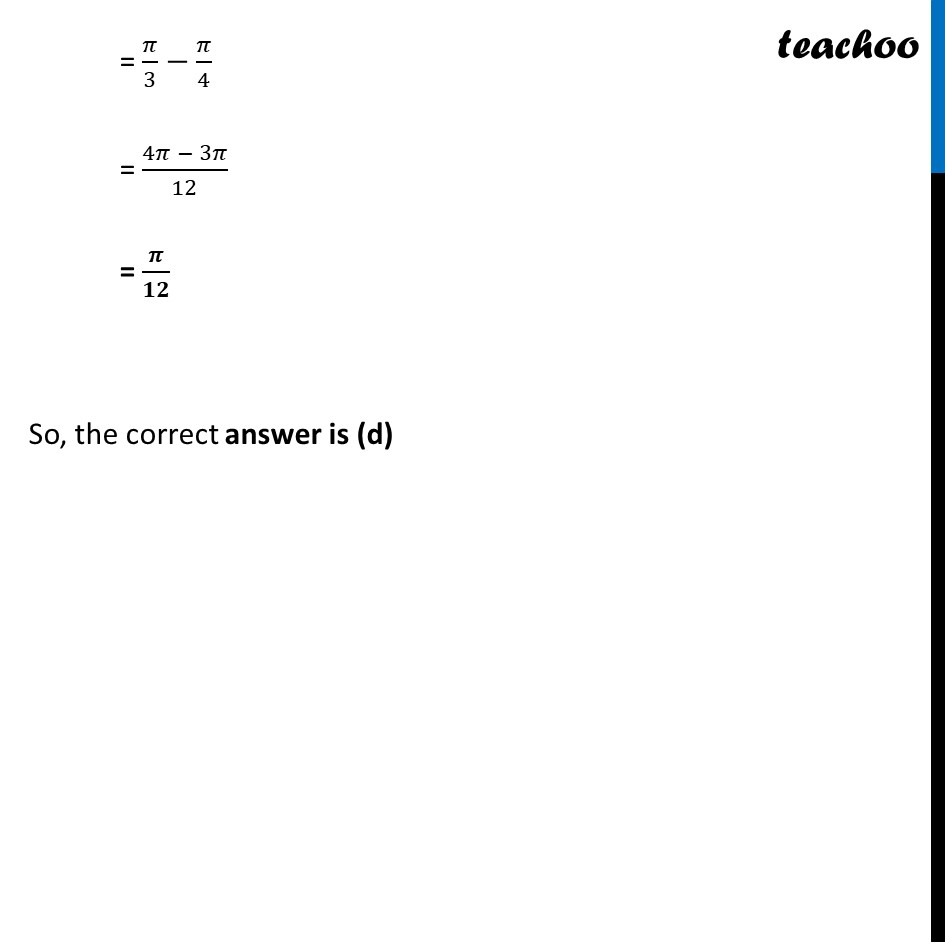## (d) 3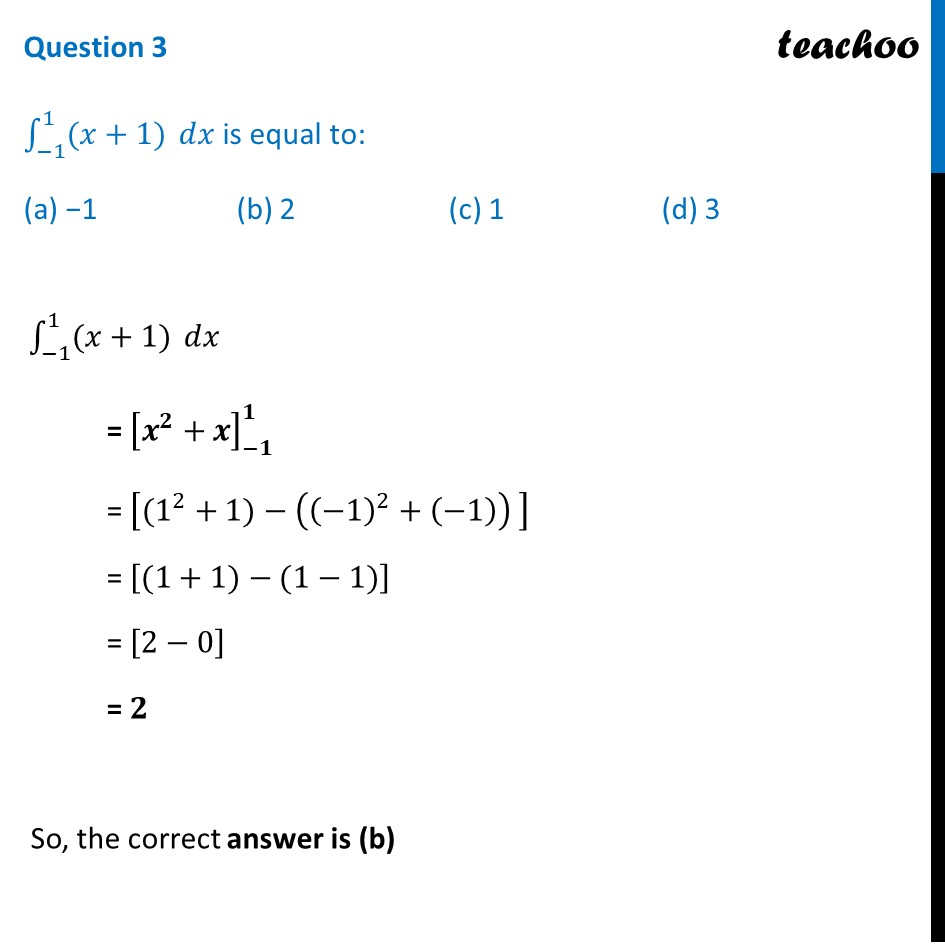## (d) log(2)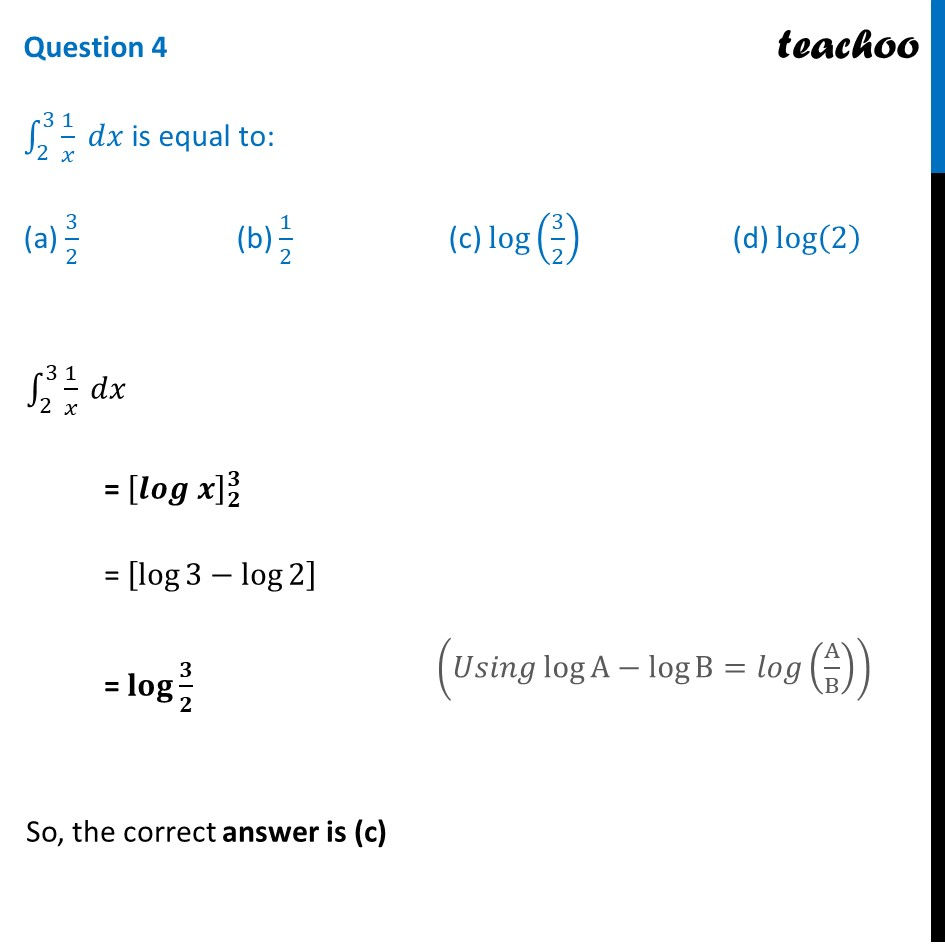## (d) e 5 - e 4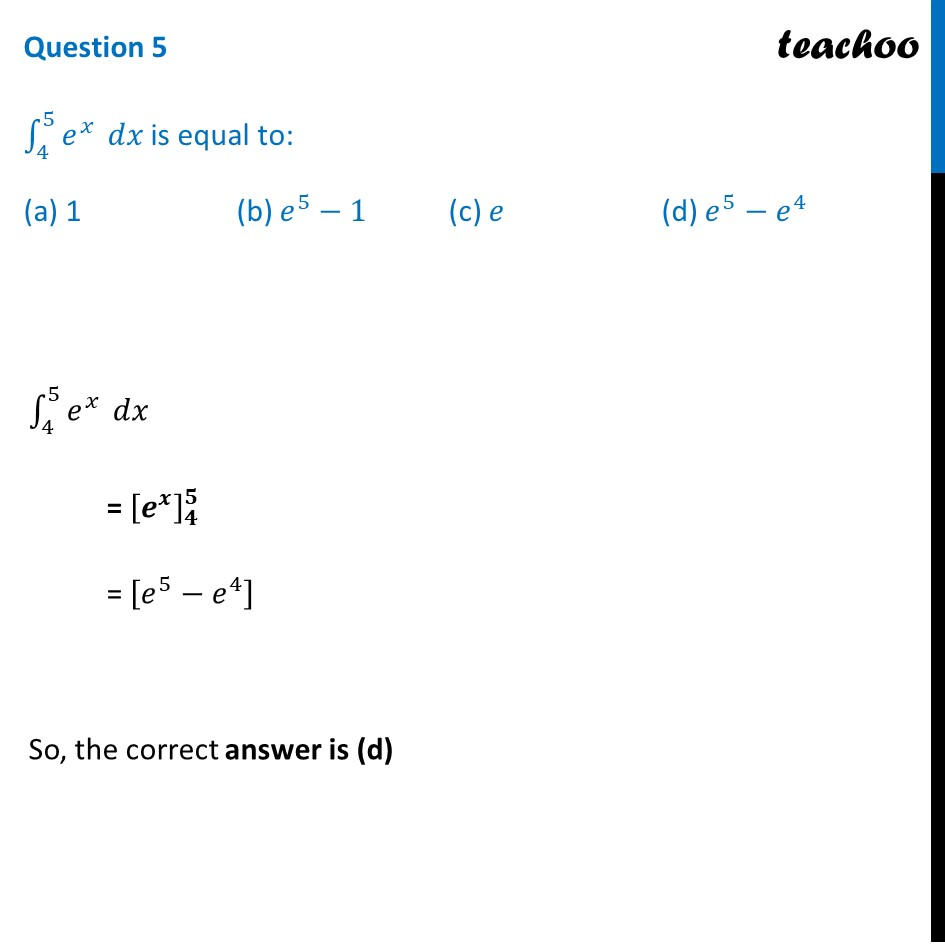Get live Maths 1-on-1 Classs - Class 6 to 12

### Transcript

Question 4 There are many practical applications of Definite Integration. Definite integrals can be used to determine the mass of an object if its density function is known. We can also find work by integrating a force function, and the force exerted on an object submerged in a liquid. The most important application of Definite Integration is finding the area under the curve. Let 𝑓 be a continuous function defined on the closed interval [a, b] and F be an antiderivative of 𝑓 then ∫_𝑎^𝑏▒〖𝑓(𝑥)〗 𝑑𝑥=〖[𝐹 (𝑥)]〗_𝑎^𝑏=𝐹(𝑏)−𝐹(𝑎) It is very useful because it gives us a method of calculating the definite integral more easily. There is no need to keep integration constant C because if we consider 𝐹(𝑥)+𝐶 instead of 𝐹(𝑥). We get ∫_𝑎^𝑏▒〖𝑓(𝑥)〗 𝑑𝑥=〖[𝐹 (𝑥)+𝐶]〗_𝑎^𝑏 =𝐹(𝑏)+𝐶−𝐹(𝑎)−𝐶 =𝐹(𝑏)−𝐹(𝑎) Based on the above information, answer the following questions. Question 1 ∫_2^3▒𝑥^2 𝑑𝑥 is equal to: (a) 7/3 (b) 9 (c) 19/3 (d) 1/3 ∫_2^3▒𝑥^2 𝑑𝑥 = [𝒙^𝟑/𝟑]_𝟐^𝟑 =[3^3/3−2^3/3] =[(27 − 8)/3] = 𝟏𝟗/𝟑 So, the correct answer is (c) Question 2 ∫_1^(√3)▒𝑑𝑥/(1 + 𝑥^2 ) is equal to: (a) 𝜋/3 (b) 2𝜋/3 (c) 𝜋/6 (d) 𝜋/12 ∫_1^(√3)▒𝑑𝑥/(1 + 𝑥^2 ) = [1/1 𝑡𝑎𝑛^(−1) 𝑥]_1^√3 = [𝒕𝒂𝒏^(−𝟏) 𝒙]_𝟏^√3 = 𝑡𝑎𝑛^(−1) √3−𝑡𝑎𝑛^(−1) 1 = 𝜋/3−𝜋/4 = (4𝜋 − 3𝜋)/12 = 𝝅/𝟏𝟐 So, the correct answer is (d) Question 3 ∫_(−1)^1▒〖(𝑥+1)〗 𝑑𝑥 is equal to: (a) −1 (b) 2 (c) 1 (d) 3 ∫_(−1)^1▒〖(𝑥+1)〗 𝑑𝑥 = [𝒙^𝟐+𝒙]_(−𝟏)^𝟏 = [(1^2+1)−((−1)^2+(−1)) ] = [(1+1)−(1−1)] = [2−0] = 𝟐 So, the correct answer is (b) Question 4 ∫_2^3▒1/𝑥 𝑑𝑥 is equal to: (a) 3/2 (b) 1/2 (c) log(3/2) (d) log(2) ∫_2^3▒1/𝑥 𝑑𝑥 = [𝒍𝒐𝒈 𝒙]_𝟐^𝟑 = [log⁡3−log⁡2 ] = 𝐥𝐨𝐠⁡〖𝟑/𝟐〗 So, the correct answer is (c) Question 5 ∫_4^5▒𝑒^𝑥 𝑑𝑥 is equal to: (a) 1 (b) 𝑒^5−1 (c) 𝑒 (d) 𝑒^5−𝑒^4 ∫_4^5▒𝑒^𝑥 𝑑𝑥 = [𝒆^𝒙 ]_𝟒^𝟓 = [𝑒^5−𝑒^4 ] So, the correct answer is (d)